Please keep in mind, that DALEXtra now support usage of mlr with dedicated `explain_mlr` function. It is recommended to use it.

# 1 Introduction

DALEX is designed to work with various black-box models like tree ensembles, linear models, neural networks etc. Unfortunately R packages that create such models are very inconsistent. Different tools use different interfaces to train, validate and use models.

In this vignette we will show explanations for models from mlr (Bischl et al. 2016).

# 2 Regression use case - apartments data

``````library(DALEX)
library(mlr)
library(breakDown)``````

To illustrate applications of DALEX to regression problems we will use an artificial dataset `apartments` available in the DALEX package. Our goal is to predict the price per square meter of an apartment based on selected features such as construction year, surface, floor, number of rooms, district. It should be noted that four of these variables are continuous while the fifth one is a categorical one. Prices are given in Euro.

``````data(apartments)
``````##   m2.price construction.year surface floor no.rooms    district
## 1     5897              1953      25     3        1 Srodmiescie
## 2     1818              1992     143     9        5     Bielany
## 3     3643              1937      56     1        2       Praga
## 4     3517              1995      93     7        3      Ochota
## 5     3013              1992     144     6        5     Mokotow
## 6     5795              1926      61     6        2 Srodmiescie``````

## 2.1 The explain() function

The first step of using the DALEX package is to wrap-up the black-box model with meta-data that unifies model interfacing.

In this vignette we will use 3 models: random forest, gradient boosting machine model, and neutral network for regression.

According to the semantics of the mlr package at the beginning we have to make our regression task using function `makeRegrTask()` and build learners for our models using the `makeLearner()` function.

``````set.seed(123)
regr_lrn_rf <- makeLearner("regr.randomForest")
regr_lrn_nn <- makeLearner("regr.nnet")
regr_lrn_gbm <- makeLearner("regr.gbm", par.vals = list(n.trees = 500))``````

Additionally, for the neural network model we set additional parameters and do the data preprocessing.

``````regr_lrn_nn <- setHyperPars(regr_lrn_nn, par.vals = list(maxit=500, size=2))
regr_lrn_nn <- makePreprocWrapperCaret(regr_lrn_nn, ppc.scale=TRUE, ppc.center=TRUE)``````

Below, we use the mlr function `train()` to fit our models.

``````regr_rf <- train(regr_lrn_rf, regr_task)

To create an explainer for these models it is enough to use `explain()` function with the model, data and y parameters. Validation dataset for the models is `apartmentsTest` data from the DALEX package. For the models created by mlr package we have to provide custom predict function which takes two arguments: model and newdata and returns a numeric vector with predictions because function `predict()` from mlr returns not only predictions but an object with more information.

``````data(apartmentsTest)
custom_predict <- function(object, newdata) {pred <- predict(object, newdata=newdata)
response <- pred\$data\$response
return(response)}

explainer_regr_rf <- DALEX::explain(regr_rf, data=apartmentsTest, y=apartmentsTest\$m2.price, predict_function = custom_predict, label="rf", colorize = FALSE)``````
``````## Preparation of a new explainer is initiated
##   -> model label       :  rf
##   -> data              :  9000  rows  6  cols
##   -> target variable   :  9000  values
##   -> data              :  A column identical to the target variable `y` has been found in the `data`.  (  WARNING  )
##   -> data              :  It is highly recommended to pass `data` without the target variable column
##   -> predict function  :  custom_predict
##   -> predicted values  :  numerical, min =  1957.9 , mean =  3507.173 , max =  5796.648
##   -> residual function :  difference between y and yhat (  default  )
##   -> residuals         :  numerical, min =  -720.3678 , mean =  4.350737 , max =  1244.949
##   -> model_info        :  package Model of class: WrappedModel package unrecognized , ver. Unknown , task regression (  default  )
##   A new explainer has been created!``````
``````explainer_regr_nn <- DALEX::explain(regr_nn, data=apartmentsTest, y=apartmentsTest\$m2.price,
predict_function = custom_predict, label="nn", colorize = FALSE)``````
``````## Preparation of a new explainer is initiated
##   -> model label       :  nn
##   -> data              :  9000  rows  6  cols
##   -> target variable   :  9000  values
##   -> data              :  A column identical to the target variable `y` has been found in the `data`.  (  WARNING  )
##   -> data              :  It is highly recommended to pass `data` without the target variable column
##   -> predict function  :  custom_predict
##   -> predicted values  :  numerical, min =  3042.321 , mean =  3490.524 , max =  3754.98
##   -> residual function :  difference between y and yhat (  default  )
##   -> residuals         :  numerical, min =  -2036.98 , mean =  20.99976 , max =  2924.02
##   -> model_info        :  package Model of class: PreprocModel package unrecognized , ver. Unknown , task regression (  default  )
##   A new explainer has been created!``````
``````explainer_regr_gbm <- DALEX::explain(regr_gbm, data=apartmentsTest, y=apartmentsTest\$m2.price,
predict_function = custom_predict, label="gbm", colorize = FALSE)``````
``````## Preparation of a new explainer is initiated
##   -> model label       :  gbm
##   -> data              :  9000  rows  6  cols
##   -> target variable   :  9000  values
##   -> data              :  A column identical to the target variable `y` has been found in the `data`.  (  WARNING  )
##   -> data              :  It is highly recommended to pass `data` without the target variable column
##   -> predict function  :  custom_predict
##   -> predicted values  :  numerical, min =  1643.091 , mean =  3509.271 , max =  6635.908
##   -> residual function :  difference between y and yhat (  default  )
##   -> residuals         :  numerical, min =  -369.3323 , mean =  2.252383 , max =  492.8315
##   -> model_info        :  package Model of class: WrappedModel package unrecognized , ver. Unknown , task regression (  default  )
##   A new explainer has been created!``````

## 2.2 Model performance

Function `model_performance()` calculates predictions and residuals for validation dataset.

``````mp_regr_rf <- model_performance(explainer_regr_rf)
mp_regr_gbm <- model_performance(explainer_regr_gbm)
mp_regr_nn <- model_performance(explainer_regr_nn)``````

Generic function `print()` returns quantiles for residuals.

``mp_regr_rf``
``````##           0%          10%          20%          30%          40%
## -1244.948784  -398.788140  -198.604201   -88.250267    -7.442933
##          50%          60%          70%          80%          90%
##    53.924498   105.918427   158.327152   216.723678   292.280918
##         100%
##   720.367763``````

Generic function `plot()` shows reversed empirical cumulative distribution function for absolute values from residuals. Plots can be generated for one or more models.

``plot(mp_regr_rf, mp_regr_nn, mp_regr_gbm)``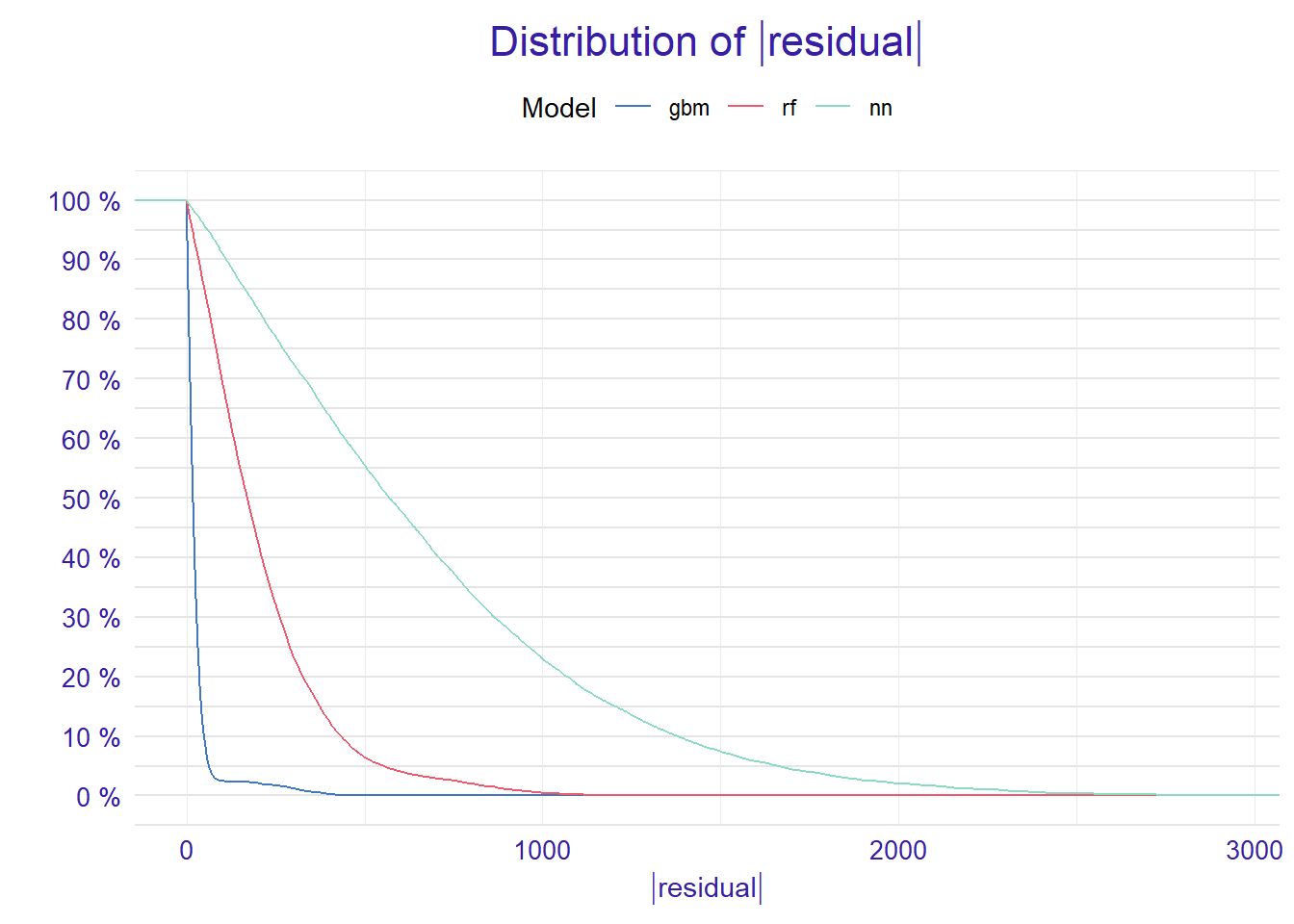The figure above shows that majority of residuals for random forest are smaller than residuals for the neural network and gbm.

We are also able to use the `plot()` function to get an alternative comparison of residuals. Setting the `geom = "boxplot"` parameter we can compare the distribution of residuals for selected models.

``plot(mp_regr_rf, mp_regr_nn, mp_regr_gbm, geom = "boxplot")``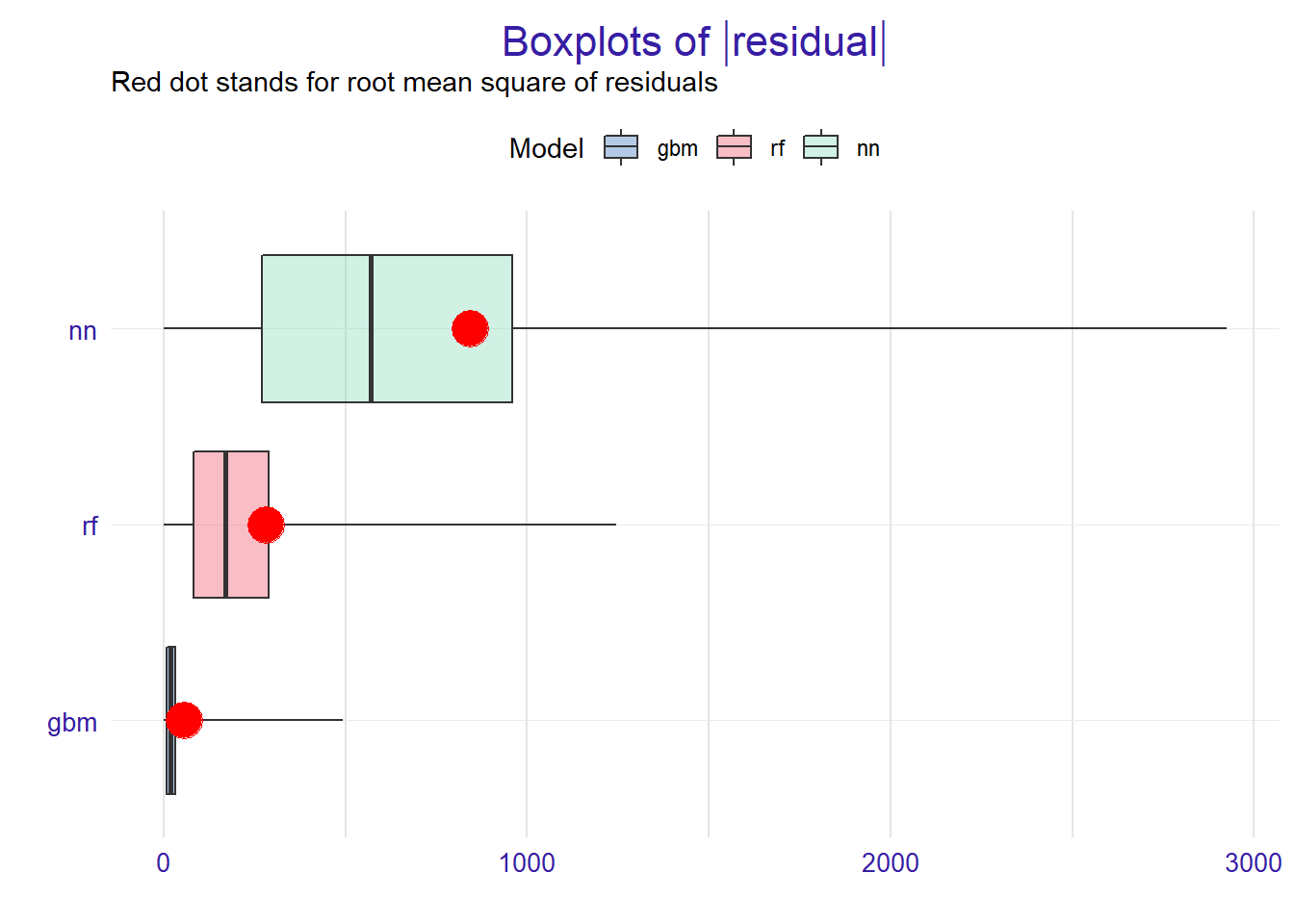## 2.3 Variable importance

Using he DALEX package we are able to better understand which variables are important.

Model agnostic variable importance is calculated by means of permutations. We simply substract the loss function calculated for validation dataset with permuted values for a single variable from the loss function calculated for validation dataset.

This method is implemented in the `variable_importance()` function.

``````vi_regr_rf <- variable_importance(explainer_regr_rf, loss_function = loss_root_mean_square)
vi_regr_gbm <- variable_importance(explainer_regr_gbm, loss_function = loss_root_mean_square)
vi_regr_nn <- variable_importance(explainer_regr_nn, loss_function = loss_root_mean_square)``````

We can compare all models using the generic `plot()` function.

``plot(vi_regr_rf, vi_regr_gbm, vi_regr_nn)``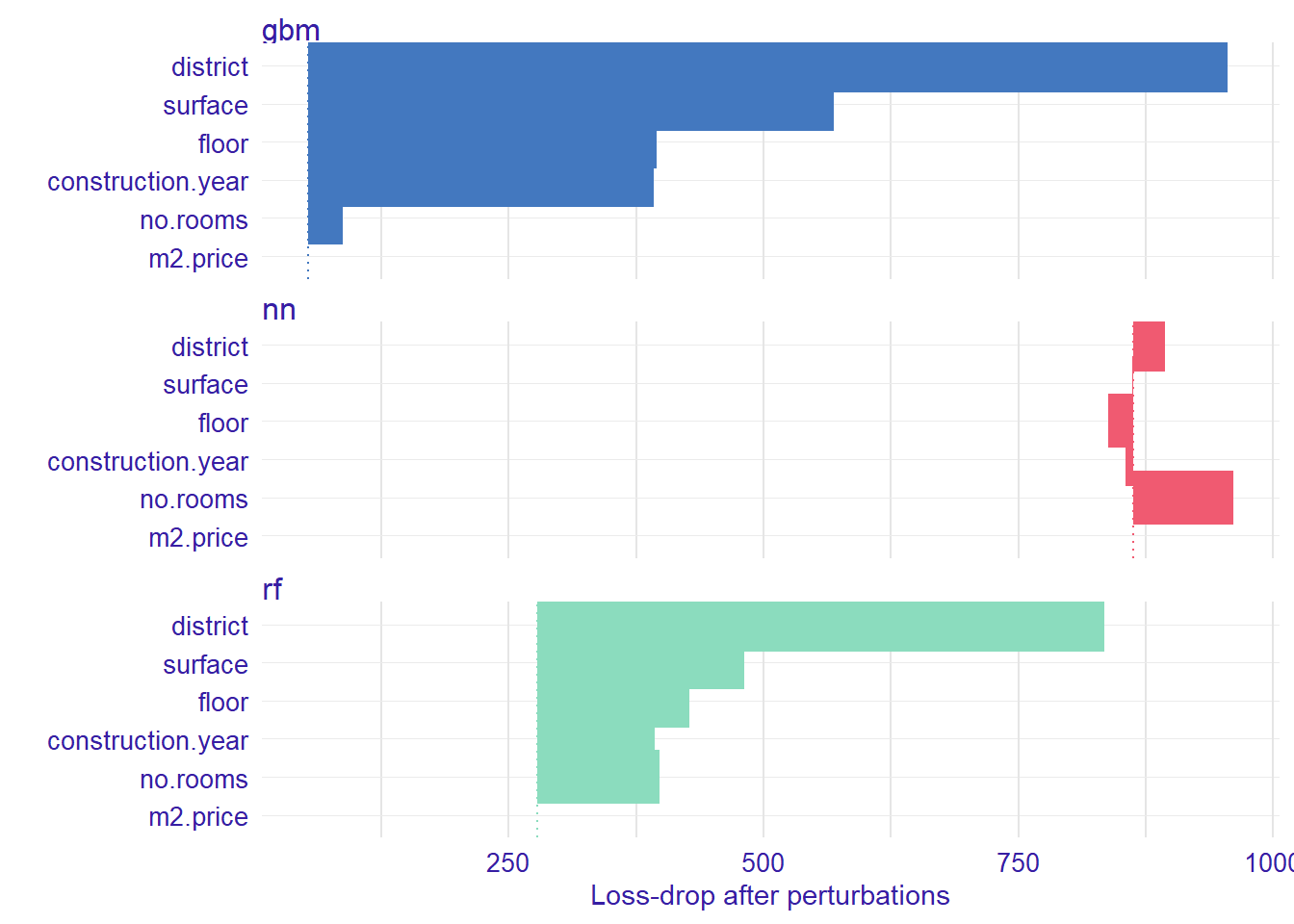Length of the interval coresponds to a variable importance. Longer interval means larger loss, so the variable is more important.

For better comparison of the models we can hook the variabe importance at 0 using the `type=difference`.

``````vi_regr_rf <- variable_importance(explainer_regr_rf, loss_function = loss_root_mean_square, type="difference")
vi_regr_gbm <- variable_importance(explainer_regr_gbm, loss_function = loss_root_mean_square, type="difference")
vi_regr_nn <- variable_importance(explainer_regr_nn, loss_function = loss_root_mean_square, type="difference")

plot(vi_regr_rf, vi_regr_gbm, vi_regr_nn)``````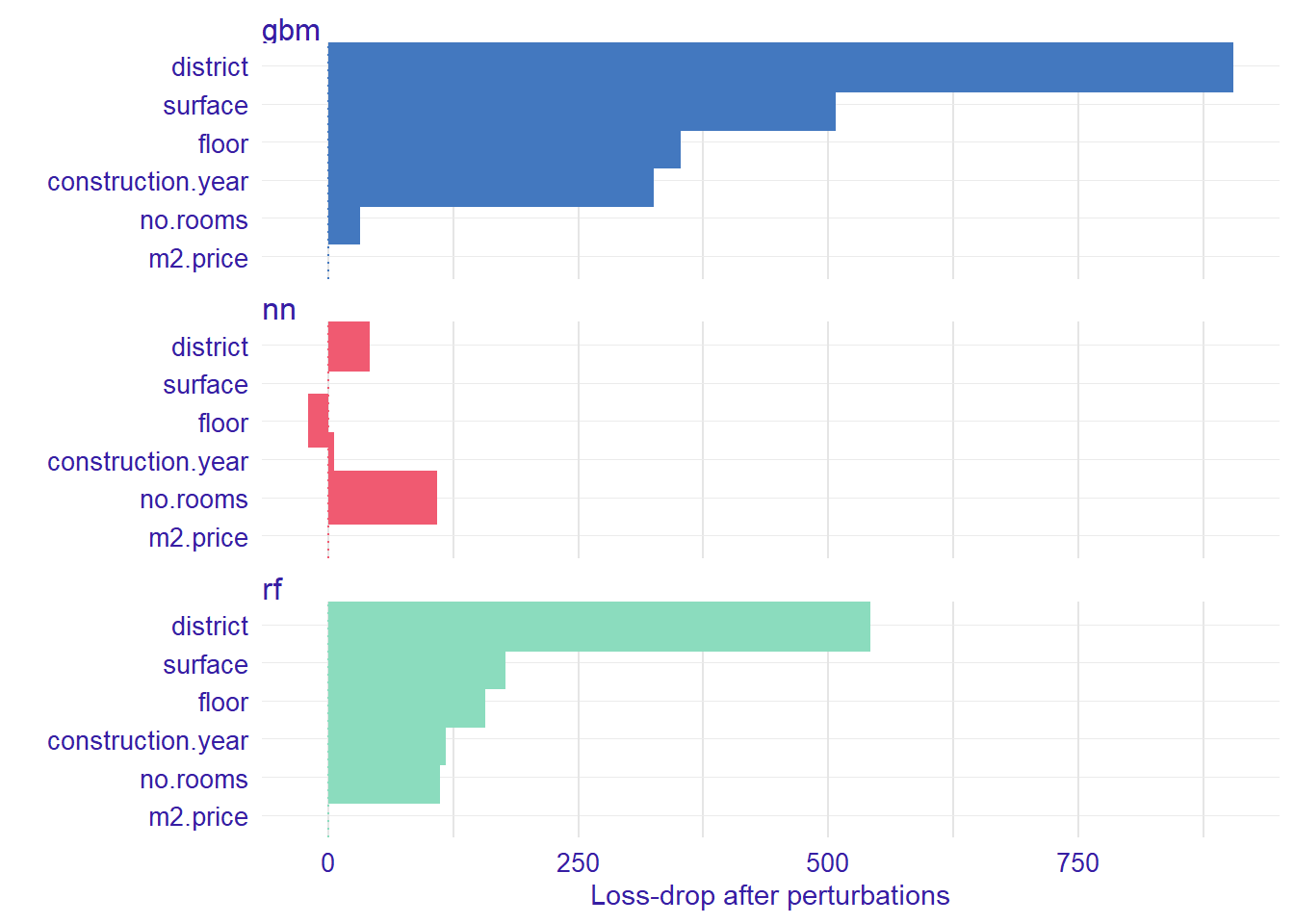We see that in random forest and gbm model the most important variable is `district`.

## 2.4 Variable response

Explainers presented in this section are designed to better understand the relation between a variable and model output.

For more details of methods desribed in this section see Variable response section in DALEX docs.

### 2.4.1 Partial Dependence Plot

Partial Dependence Plots (PDP) are one of the most popular methods for exploration of the relation between a continuous variable and the model outcome.

Function `variable_response()` with the parameter `type = "pdp"` calls `pdp::partial()` function to calculate PDP response.

``````pdp_regr_rf  <- variable_response(explainer_regr_rf, variable =  "construction.year", type = "pdp")
pdp_regr_gbm  <- variable_response(explainer_regr_gbm, variable =  "construction.year", type = "pdp")
pdp_regr_nn  <- variable_response(explainer_regr_nn, variable =  "construction.year", type = "pdp")

plot(pdp_regr_rf, pdp_regr_gbm, pdp_regr_nn)``````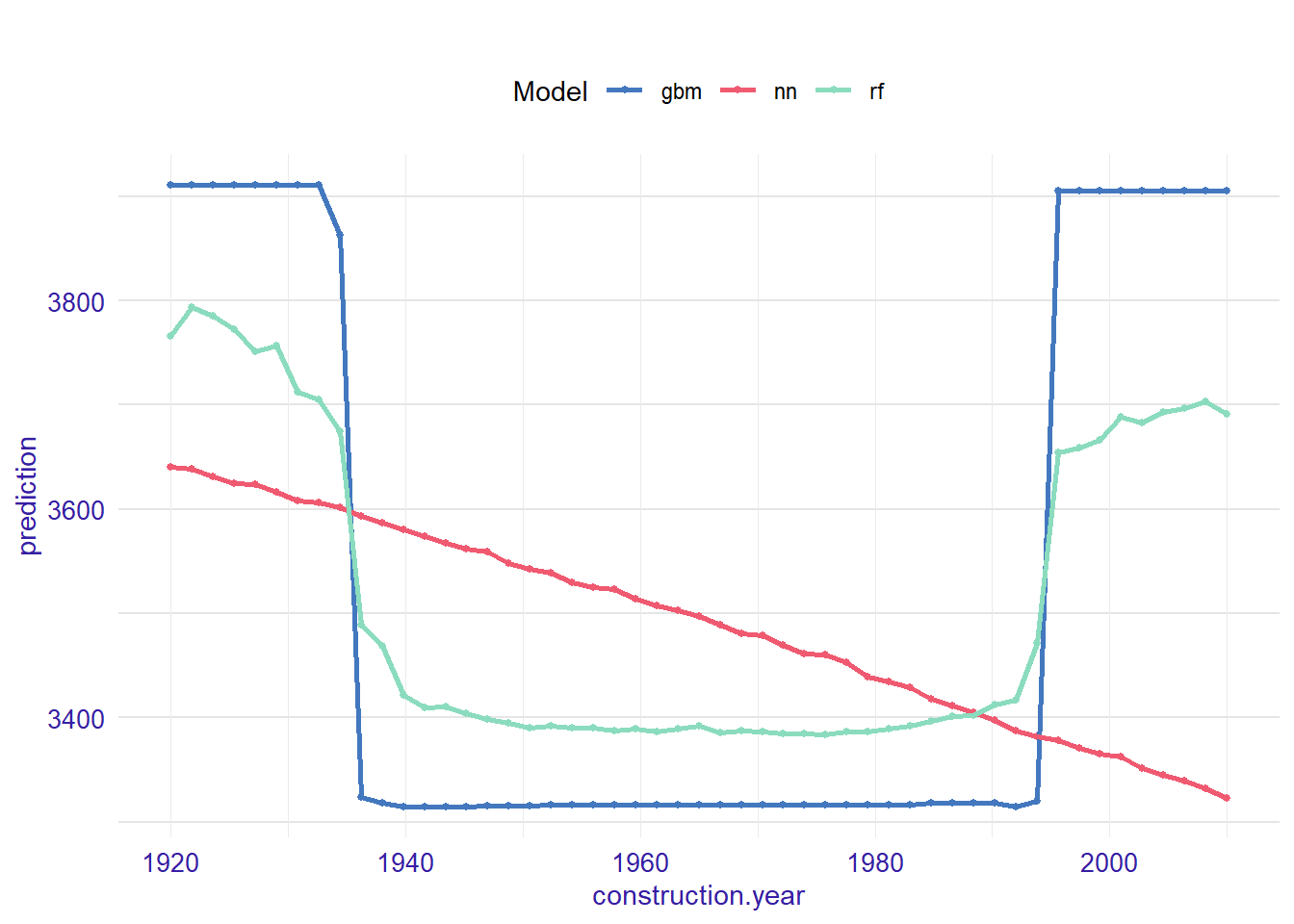We use PDP plots to compare our 3 models. As we can see above performance of random forest may tell us that we have non-linear relation in the data. It looks like the neural network and gbm don’t captured that relation.

### 2.4.2 Acumulated Local Effects plot

Acumulated Local Effects (ALE) plot is the extension of PDP, that is more suited for highly correlated variables.

Function `variable_response()` with the parameter `type = "ale"` calls `ALEPlot::ALEPlot()` function to calculate the ALE curve for the variable `construction.year`.

``````ale_regr_rf  <- variable_response(explainer_regr_rf, variable =  "construction.year", type = "ale")
ale_regr_gbm  <- variable_response(explainer_regr_gbm, variable =  "construction.year", type = "ale")
ale_regr_nn  <- variable_response(explainer_regr_nn, variable =  "construction.year", type = "ale")

plot(ale_regr_rf, ale_regr_gbm, ale_regr_nn)``````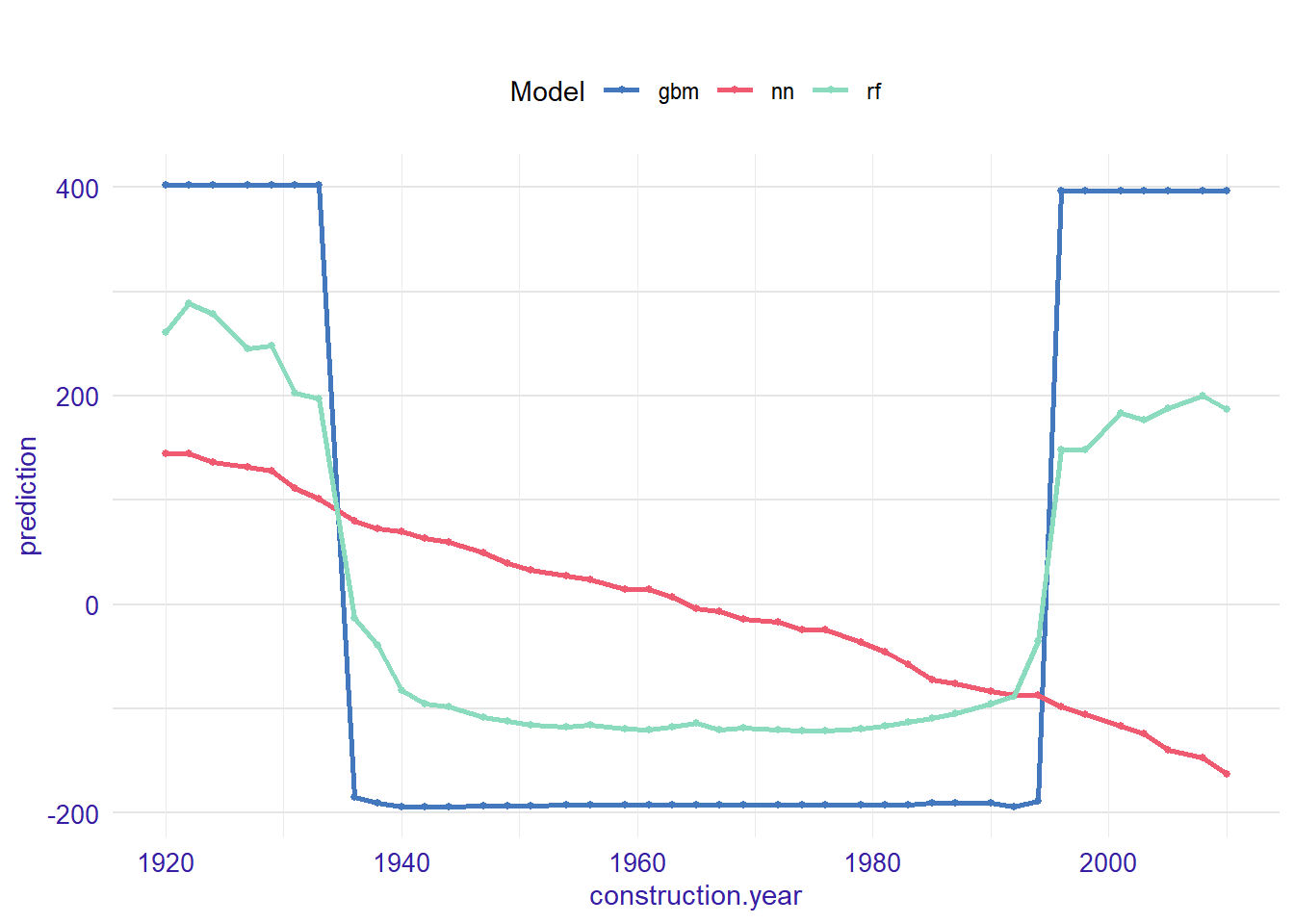### 2.4.3 Merging Path Plots

Merging Path Plot is a method for exploration of a relation between a categorical variable and model outcome.

Function `variable_response()` with the parameter `type = "factor"` calls `factorMerger::mergeFactors()` function.

``````mpp_regr_rf  <- variable_response(explainer_regr_rf, variable =  "district", type = "factor")
mpp_regr_gbm  <- variable_response(explainer_regr_gbm, variable =  "district", type = "factor")
mpp_regr_nn  <- variable_response(explainer_regr_nn, variable =  "district", type = "factor")

plot(mpp_regr_rf, mpp_regr_gbm, mpp_regr_nn)``````